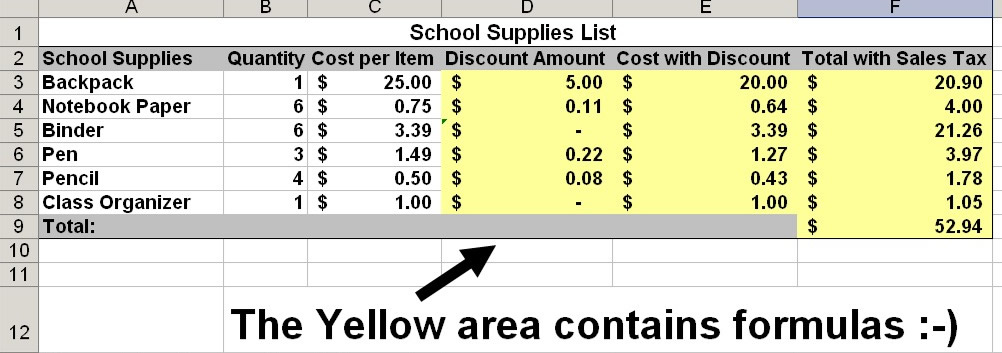# School Supplies Spreadsheet Student Activity Page

1. You need some school supplies. Here is a list of items (at regular price) that you need to purchase:
• One backpack, \$25.00 each
• Six packs of notebook paper, \$.75 each
• Six binders, \$3.39 each
• Three pens, \$1.49 each
• Four pencils, \$.50 each
• One class organizer, \$1.00 each
 Discounts on regular-priced items: Backpacks    20% off Protractors    10% off Notebook Paper  15% off Writing Utensils  15% off
1. Using a spreadsheet program (Excel), create a spreadsheet with the following columns:
• School Supplies
• Quantity
• Cost per Item
• Discount \$ Amount
• Cost with Discount
• Total with Sales Tax
2. Enter the supplies and prices from your list in the appropriate columns. Do not type the dollar sign to show monetary values. Instead, type the numbers with appropriate decimals first, and then format the cells to currency. (Since not every item has a discount, you will need to enter 0% in the formula of non-discounted items)
3. Use the appropriate formulas to calculate the “Discount \$ Amount”, “Cost with Discount” and “Total with Sales Tax” for each item. Use cell names in your formulas instead of actual numbers.
4. Assume that the sales tax is 4.5%, and use this amount to make your final calculations.
5. Bold and center all your labels. Left align the text within the other cells.
6. Calculate the total spent (Make sure to include quantity, cost, discount, tax  – ex: 5 widgets at \$5.00 a piece with a 20% discount and 5% sales tax equals \$21.00)
7. Save the file as "Last First School Supplies"

## Example